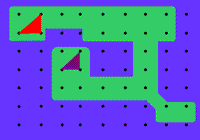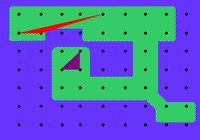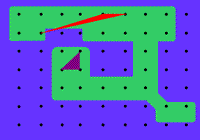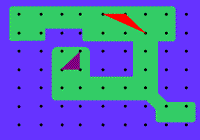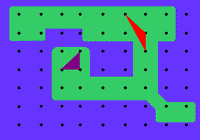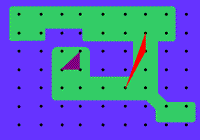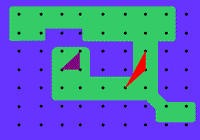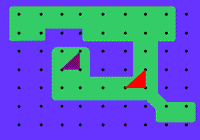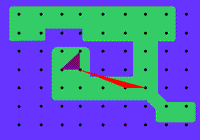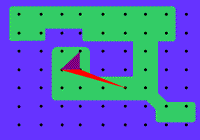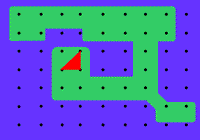#### You may also like### Uncanny Triangles

Can you help the children find the two triangles which have the lengths of two sides numerically equal to their areas?### Triangle Transformation

Start with a triangle. Can you cut it up to make a rectangle?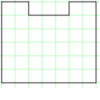### Rod Area

This task challenges you to create symmetrical U shapes out of rods and find their areas.

# Triangle Island

##### Age 7 to 11Challenge Level

Hugo sent us pictures of his solution. Well done, Hugo!

I know that the area of a triangle is given by the forumula $\frac{1}{2} \times base \times height.$ The original triangle has base $1$ and height $1$, so as long as each triangle on the way also has base $1$ and height $1$ the area will stay the same".